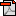# Monkeyshines

## Tree Comparison

Throughout the course of my degree I have found it useful to write summaries of the various aspects of phylogenetics and biology that I have learned. That these will be useful to others is perhaps a vain hope, in both senses of the word, but I thought I might as well publish some of them on my blog. (It also afforded me the chance to try my hand at LyX/LaTeX.) For your reading convenience, this post is available as a pdf.

Tree Comparison
Phylogenetic trees have two properties that can usefully be compared, their topologies and their branch lengths. Usually, the desired outcome of a tree comparison is a single number, indicating how different the trees are from one another. Reducing multiple complex structures to a single interpretable digit is difficult, even when just comparing two trees; a range of methods have been developed, most of which use (sometimes implicitly) graph theoretical measures of distance. Note that this is different from the situation of tree evaluation, where the aim is to determine whether some trees are a better representation of evolutionary history than others. Tree comparison is often done after evaluation, to gauge how much credence and importance to give to the results of the evaluation (there is, as yet, no method to state formally that the difference between trees is significant).

Felsenstein (2004, pp.528-535) provides a historical overview of phylogenetic tree comparison, starting with the symmetric difference metric, also known as the Robinson-Foulds (RF) distance, which measures differences in topology between a pair of (possibly multifurcating) trees (Robinson and Foulds, 1981). The symmetric difference can be conceptualised as the minimum number of transformations that are required to convert one tree to the other, where a transformation corresponds to either removing a branch and merging the nodes it connected, or by splitting a node into two and inserting a branch between the new nodes. The symmetric difference is widely used, but can be highly sensitive; that is, it can have a high value for trees which are intuitively similar (Felsenstein, 2004).

Including information on branch lengths in tree comparisons is potentially useful, particularly when the tree has a relatively wide range of branch lengths. The weighted Robinson-Foulds distance (Robinson and Foulds, 1979) and the branch score (Kuhner and Felsenstein, 1994) are two metrics that use branch length information, and both are based on the symmetric difference. The weighted RF distance is the sum of the differences between corresponding branch lengths; a branch length is considered to be zero if it does not exist in one of the trees. The branch score is similar, but squares the differences before adding them, and the square root of this sum is named the branch-length distance (BLD) (Felsenstein, 2004).

The pair of trees being compared can be mapped to two points in tree space, which suggests another distance metric, the geodesic distance, defined as the shortest path between two points in tree space. In tree space, the weighted RF distance and the BLD correspond to Manhattan and Euclidean distances, respectively (Kupczok et al., 2008). Calculating the geodesic distance may be computationally prohibitive for large trees, but good approximations are available (Kupczok et al., 2008).

All of the distances that use branch lengths will produce relatively high values if the branches in one tree tend to be larger, even if the topologies are very similar; that is, if the evolutionary rate differs between the trees. This behaviour may or may not be desirable, so to prevent differences in rate from having a disproportionate effect, Kuhner and Felsenstein (1994) suggested using relative branch lengths, dividing each branch length by the sum of all branch lengths. As far as I am aware, this has not been implemented in any publicly available software. The K score is a modification of the BLD that scales one tree to have similar global divergence to the other before calculating the BLD, but the scaling means that the K score is no longer mathematically defined as a distance, and its use is not always appropriate (Soria-Carrasco et al., 2007).

Citing this Document
[If referring to this document, please cite its location on the Monkeyshines website: http://www.monkeyshines.co.uk/blog/archives/891]

References

• Felsenstein, J. (2004) Inferring Phylogenies. Sinauer, Sunderland, Massachusetts.
• Kuhner,M.K. and Felsenstein, J. (1994) A simulation comparison of phylogeny algorithms under equal and unequal evolutionary rates. Molecular Biology and Evolution, 11, 459-468. Pubmed
• Kupczok, A. et al. (2008) An exact algorithm for the geodesic distance between phylogenetic trees. Journal of Computational Biology, 15, 577-591. Pubmed
• Robinson, D.F. and Foulds, L.R. (1979) Comparison of weighted labelled trees. Lecture Notes in Mathematics, 748, 119-126.
• Robinson, D.F. and Foulds, L.R. (1981) Comparison of phylogenetic trees. Mathematical Biosciences, 53, 131-147.
• Soria-Carrasco, V. et al. (2007) The Ktree score: quantification of differences in the relative branch length and topology of phylogenetic trees. Bioinformatics, 23, 2954-2956. Pubmed

 Name (required): Email (required): (will not be published) Website: